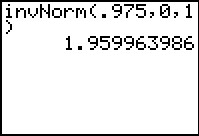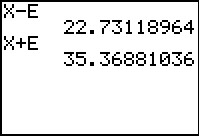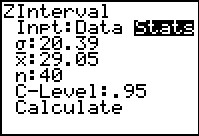# Activities

••• ##### Subject Area

• Math: Statistics: Confidence Intervals

• ##### Author9-12

15 Minutes

• ##### Device
• TI-83 Plus Family
• TI-84 Plus
• TI-84 Plus Silver Edition
•TI-84 Plus C Silver Edition
•TI-84 Plus CE
• ##### Software

TI Connect™
TI Connect™ CE

• ##### Accessories

TI Connectivity Cable

## Means With Confidence#### Activity Overview

Students estimate the true mean of a population when the standard deviation is known by finding the sample mean, margin of error and confidence interval.

#### Key Steps

•Students work through multiple-choice questions on the worksheet to review the standard normal curve and the percentiles.

The Central Limit Theorem is also reviewed and built on to enable students to construct confidence intervals when trying to estimate the mean of the population.

•Students will estimate the true mean of the longest reception for all American Football League receivers, given the standard deviation of the population.

The teacher will lead them through each step, calculating the sample mean, z-score, margin of error, and the confidence interval. Students will then interpret the confidence interval and confirm their answer with the ZInterval command.

•Students are given homework problems that ask them to find different confidence intervals using the sample data.

The true population means for the AFC receivers is also given on the teacher document so that students can check the validity of the confidence intervals.